Open in App
Not now

# Java Program To Perform Union of Two Linked Lists Using Priority Queue

• Difficulty Level : Expert
• Last Updated : 30 May, 2022

Given two linked lists, your task is to complete the function make union(), which returns the union of two linked lists. This union should include all the distinct elements only. The new list formed should be in non-decreasing order.

```Input: L1 = 9->6->4->2->3->8
L2 = 1->2->8->6->2
Output: 1 2 3 4 6 8 9```

Approach:

There are two types of heap as we all know that is min heap and max heap

• Min heap – Stores all the elements in ascending order
• Max heap – Stores all the elements in descending order

Let us first visualize using min heap in order to interpret program execution how union of two linked list is carried on, so de we are having two operations as listed to visualize:

1. Insertion
2. Removal

Insertion: After inserting all the distinct elements of two linked lists,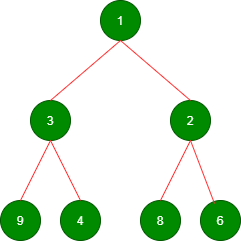Removal: Removing the root until minheap is empty

Removing the root with value 1: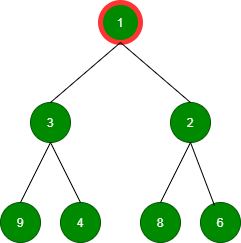Removing the root with value 2: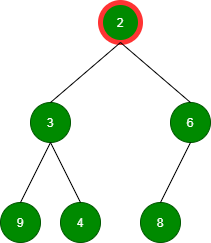Removing the root with value 3: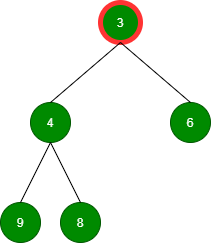Removing the root with value 4: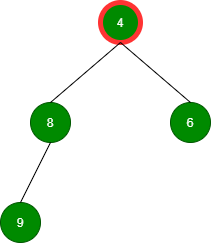Removing the root with value 6: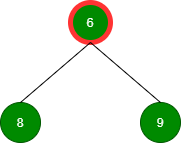Removing the root with value 8: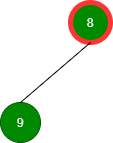Removing the root with value 9: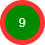Hence we can conclude that in the min-heap, the smallest element will be at the root of the heap, and in the max heap, the greatest element will be at the root of the heap. While implementing the remove() function on heap, the root element will be removed. Since the output should be in increasing order, Min heap can be used. Priority Queue is used to implement min heap in java.

Example

## Java

 `// JAva Program toIllustrate Union of Two Linked Lists``// Using Priority Queue` `// Importing basic required classes``import` `java.io.*;``import` `java.util.*;` `// Class 1``// Helper class``// Node creation``class` `Node {` `    ``// Data and addressing variable of node``    ``int` `data;``    ``Node next;` `    ``// Constructor to initialize node``    ``Node(``int` `a)``    ``{``        ``data = a;``        ``next = ``null``;``    ``}``}` `// Class 2``// Main class``public` `class` `GfG {``    ``// Reading input via Scanner class``    ``static` `Scanner sc = ``new` `Scanner(System.in);` `    ``// Method 1``    ``// To create the input list1``    ``public` `static` `Node inputList1()``    ``{``        ``// Declaring node variables that is``        ``// Head and tail``        ``Node head, tail;` `        ``// Custom input node elements` `        ``head = tail = ``new` `Node(``9``);` `        ``tail.next = ``new` `Node(``6``);``        ``// Fetching for next node``        ``// using next() method``        ``tail = tail.next;` `        ``// Similarly for node 3``        ``tail.next = ``new` `Node(``4``);``        ``tail = tail.next;` `        ``// Similarly for node 4``        ``tail.next = ``new` `Node(``2``);``        ``tail = tail.next;` `        ``//  Similarly for node 5``        ``tail.next = ``new` `Node(``3``);``        ``tail = tail.next;` `        ``// Similarly for node 6``        ``tail.next = ``new` `Node(``8``);``        ``tail = tail.next;` `        ``// Returning the head``        ``return` `head;``    ``}` `    ``// Method 2``    ``// To create the input List2``    ``// Similar to method 1 but for List2``    ``public` `static` `Node inputList2()``    ``{``        ``Node head, tail;` `        ``head = tail = ``new` `Node(``1``);` `        ``tail.next = ``new` `Node(``2``);``        ``tail = tail.next;` `        ``tail.next = ``new` `Node(``8``);``        ``tail = tail.next;` `        ``tail.next = ``new` `Node(``6``);``        ``tail = tail.next;` `        ``tail.next = ``new` `Node(``2``);``        ``tail = tail.next;` `        ``return` `head;``    ``}` `    ``// Method 3``    ``// To print the union list``    ``public` `static` `void` `printList(Node n)``    ``{``        ``// Till there is a node``        ``// condition holds true``        ``while` `(n != ``null``) {``            ``// Print the node``            ``System.out.print(n.data + ``" "``);``            ``// Moving onto next node``            ``n = n.next;``        ``}``    ``}` `    ``// Method 4``    ``// main driver method``    ``public` `static` `void` `main(String args[])``    ``{``        ``// Taking input for List 1 and List 2``        ``Node head1 = inputList1();``        ``Node head2 = inputList2();` `        ``// Calling``        ``Union obj = ``new` `Union();``        ``printList(obj.findUnion(head1, head2));``    ``}``}` `// Class 3``// To make the union of two linked list``class` `Union {``    ``public` `static` `Node findUnion(Node head1, Node head2)``    ``{``        ``// Creating a priority queue where``        ``// declaring elements of integer type``        ``PriorityQueue minheap``            ``= ``new` `PriorityQueue();``        ``// Setting heads``        ``Node l1 = head1, l2 = head2;` `        ``// For List 1``        ``// Inserting elements from linked list1 into``        ``// priority queue``        ``while` `(l1 != ``null``) {``            ``if` `(!minheap.contains(l1.data)) {``                ``minheap.add(l1.data);``            ``}``            ``// Move to next element``            ``l1 = l1.next;``        ``}` `        ``// For List 2``        ``// Inserting elements from linked list2 into``        ``// priority queue``        ``while` `(l2 != ``null``) {``            ``if` `(!minheap.contains(l2.data)) {``                ``minheap.add(l2.data);``            ``}` `            ``// Move to next element``            ``l2 = l2.next;``        ``}` `        ``Node union = ``new` `Node(``0``), start = union;` `        ``// Removing until heap is empty``        ``while` `(!minheap.isEmpty()) {``            ``Node temp = ``new` `Node(minheap.remove());` `            ``// Using temp to store start``            ``start.next = temp;``            ``start = start.next;``        ``}` `        ``// Returning node``        ``return` `union.next;``    ``}``}`

Output

`1 2 3 4 6 8 9 `

Time complexity: O(nlogn), Space complexity: O(n)

My Personal Notes arrow_drop_up